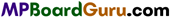These MP Board Class 11th Chemistry Notes for Chapter 6 Thermodynamics help students to get a brief overview of all the concepts.

## MP Board Class 11th Chemistry Notes Chapter 6 Thermodynamics

→ Energy : Capacity to do work is Energy.

→ Chemical energetics : Branch of chemistry which deals with the energy changes associated with chemical reaction is called chemical energetics.

→ In chemical reaction energy is used in breaking the bonds of reactant compounds whereas energy is released in the making of new bonds in product compounds.→ Exothermic reactions : Reactions in which energy is released.
A + B → C + D,+ Q or – ΔH

→ Endothermic reactions : Reactions in which energy is absorbed.
A + B → C + D,- Q or + Δ H

→ Internal energy is the sum of various types of energies of molecules of a substance. It is a state function.

→ Internal energy change (Δ E): Heat given to a system at constant temperature and constant volume is equal to increase in its Internal Energy (ΔE = qv).

→ Enthalpy (H) : Enthalpy of any system is the sum of internal energy and product of pressure and volume, i.e., H = E + PV.

→ Enthalpy change (ΔH) : Enthalpy change is the difference of enthalpies of products and reactants in a chemical reaction, i.e.,
ΔH = H(products) – H(reactants)

Enthalpy change is also related with change in internal energy and work done, i.e.,
ΔH = ΔE + PΔVHeat (qp) given to the system at constant pressure is equal to increase in enthalpy of the system ΔH = qp

→ First law of thermodynamics : According to it, Total energy of the universe is always con¬served. Mathematically AE = q + W

→ Heat of reaction : Is the enthalpy change in a chemical reaction (Difference in Enthalpy of products and reactants).

→ Heat of neutralization : Energy produced in neutralization of one gram equivalent of acid by one gram equivalent of base at constant temperature.
For a strong acid and strong base value of ΔH = -57.1 kJ.

→ Heat of combustion: At constant pressure, when one mole of any substance completely reacts with excess of oxygen, energy released in this process is called heat of combustion.

→ Heat of formation: When 1 mole of substance is formed from its constituent elements in their stable states, the energy absorbed or released is called heat of formation.

→ Heat of fusion : Enthalpy change when one mole of solid substance is liquified, is called heat
of fusion (Hfussion)

→ Heat of vaporization : When one mole liquid substance changes into gaseous state, enthalpy change in this process is called heat of vaporization (Hvap)

→ Hess’s law: The enthalpy change in a chemical process is always same whether the process is carried out in one or in several steps.→ Bond energy: The average energy required to break the bonds present in 1 mole of a gaseous molecule is called bond energy.

→ Endothermic Reaction : Absorption of heat in a reaction ΔH = +Ve .
→ Enthalpy (H) is a state function :
H = E + PV
ΔH = ΔE + PΔV .
qp, =ΔH
ΔH = ΔE + ΔnRT
gv = ΔE
4.18 x 107 Erg = 1 calorie

→ Entropy change (ΔS) = $$\frac{q_{\mathrm{rev}}}{\mathrm{T}}$$

→ For spontaneous process, ΔG is negative.

→ If the process is in equilibrium, ΔS is zero.

→ Free energy, G = H-TS and AG = ΔH-TΔS.

→ Heat of reaction at constant volume : Heat of reaction at constant volume is equal to the difference of internal energy of products (EP) and internal energy of reactants (ER). /.e.,
ΔE = Ep – ER = qv→ Heat of reaction at constant pressure (qp) : Heat of reaction at constant pressure is equal to the heat absorbed or evolved when all moles of reactants react completely at constant pressure.